# Reducing Radiated EMI for the CISPR32-Compliant Nanopower Buck Converter with the MAX38643

### Abstract

This application note explains how to reduce the radiated EMI emission in the MAX38643 nanopower buck converter. It also explains the sources of EMI noise, and provides a few simple methods to reduce the radiated EMI and make the MAX38643 buck converter compliant to the CISPR32 standard Class B limit.

#### Introduction

A high-frequency switching power supply generates electromagnetic interference (EMI) during operations, which interrupts the surrounding equipment. These EMI interferences can be either conducted or radiated in nature. The importance of the EMC in electronic equipment is increasing. More standards are accordingly emerging to limit the radiated interference generated from an electronic device.

The MAX38640–MAX38643 are the nanopower family of ultra-low quiescent current DC-DC buck converters with MOSFETs and control circuit integrated within the chip. This buck converter family operates from the 1.8V to 5.5V input voltage. It supports load currents up to 175mA, 350mA, and 700mA, with peak efficiencies of 96% and over 88% efficiency at 10µA load current.

FeaturesEfficiency
• Extends battery life
• 330nA ultra-low quiescent supply current
• 5nA Shutdown Current
• 96% Peak Efficiency and Over 88% at 10µA
• Protects system in multiple use cases:
• Reverse-Current Blocking in Shutdown
• Optional Active Discharge Feature
• Output short-circuit protection
• High efficiency:
• 96% peak efficiency
• 88% efficiency at 10 µA load current
• Reduces Size and Increases Reliability:
• 2mm x 2mm 6-pin
• 1.42mmx0.89mm, 2x3 WLP 0.4mm Pitch 6-pinFigure 1. MAX38643 Efficiency Vs Load current (VOUT = 1.8V).

#### Application circuitFigure 2. Application circuit of MAX38640.

CISPR32 is the EMI emission standard for multimedia equipment having a rated RMS AC voltage or DC supply voltage not exceeding 600V. Products for use in residential/domestic environment must comply with the Class B limit. Products for commercial use must comply with the Class A limit.

The following are the limits of radiated emission according to the CISPR32 standard for both 3-meter and 10-meter testing.

Table 1: CISPR32 Standard QPK Limits for Radiated Emission

Frequency range (MHz) 3m distance limit 10m distance limit
Class A (dBµV/m) Class B (dBµV/m) Class A (dBµV/m) Class B (dBµV/m)
30 to 230 50.5 40.5 40 30
230 to 1000 57.5 47.5 47 37

Figure 3 shows the radiated emission test setup at the laboratory. The EUT is placed on a 360° rotatable test table, which is at a 10m distance from the receiver antenna. The receiver antenna picks the EMI noise signals generating from the EUT (MAX38643) at different heights (1m to 4m). It generates the EMI peak plot from 30MHz to 1GHz frequency in the spectrum analyzer/EMI receiver.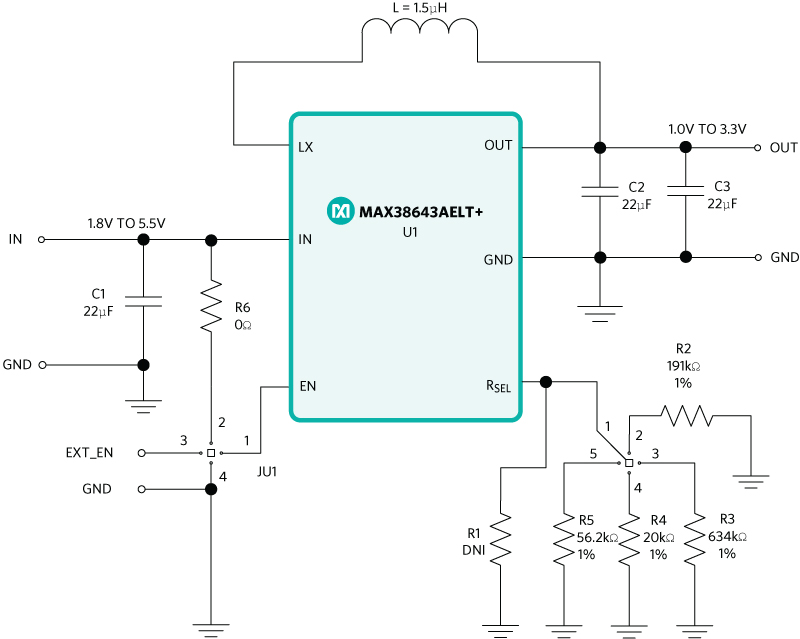Figure 3. Radiated emission measurement test setup.

#### Measurement and Layout Design to Optimize the EMI Emission

The MAX38643AELT+ buck converter was used here. It has a peak inductor current limit of 1A and can support a load current of 700mA in the µDFN package. Figure 4 is the schematic of MAX38643.Figure 4. Schematic of the MAX38643 buck converter.

#### The use case for this study is as follows

Input: Li-Ion battery with voltage range of 3.0V to 4.2V
Output voltage: 1.8V

The switches, Q1 and Q2, operate alternately in the MAX38643 buck converter. Switch Q1 is ON when Q2 is OFF, and vice versa. The current flows from CIN to the load through the inductor L and the inductor stores the energy when Q1 is ON. The switch Q2 is OFF during this period. Figure 5 shows the current path during this operation in red. The inductor discharges through the load and COUT when Q2 turns ON and Q1 turns OFF. Figure 5 shows the current path in green. The output voltage is the average of the switching voltage appearing across Q2.

The high frequency switching of Q1 and Q2 creates pulsating current with high di/dt and generates high EMI noise. This current path becomes critical for controlling the radiated EMI emission. So, a normal way to minimize the EMI emission is to reduce the area of these switching paths.Figure 5. Current paths of the buck converter based on the Q1 and Q2 switching.

This application note considers two different board layouts.

#### Board-1 PCB layout

Figures 6 and 7 show the board-1 PCB layout of MAX38643 and the EMI emission peak plot.Figure 6. PCB layout of the MAX38643 board-1 EV kit.Figure 7. Radiated emission peak plot of the MAX38643 board-1 EV kit layout.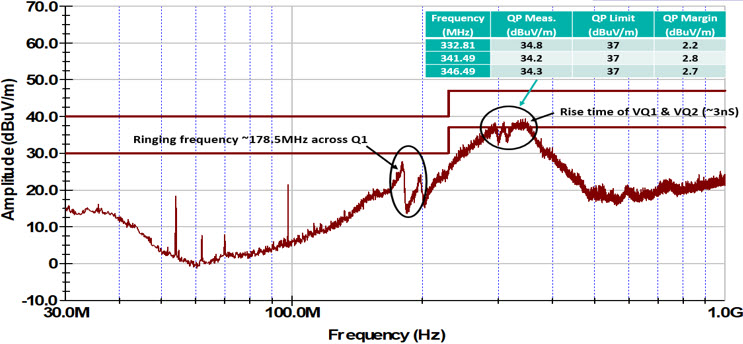Figure 8. Voltage across the switch Q1 of the MAX38643 board-1 EV kit layout.

The board-1 layout passes the CISPR32 Class B limit with a 2.2dBµV/m margin, which is very less. The EMI plot in Figure 7 has one peak at 178.5MHz, which corresponds to the ringing frequency of the parasitic components in Figure 8. The other peak is around 330MHz, which corresponds to the rise time of the voltage across Q1 and Q2.

The parasitic inductance comes into picture and creates the voltage ringing across Q1 (Figure 8). It generates the EMI noise if the CIN capacitor is far from Q1 (Figure 6). It is very important to place the CIN capacitor as near as possible to Q1.

#### Board-2 PCB layout

The CIN can be moved still closer to the IC (Figure 6) to reduce the parasitic inductance between CIN and the IC MAX38643. The CIN is placed approximately 1mm closer to the IC MAX38643 in the board-2 layout to reduce the EMI emission. Figures 9 and 10 show the board-2 PCB layout and radiated EMI emission peak plot.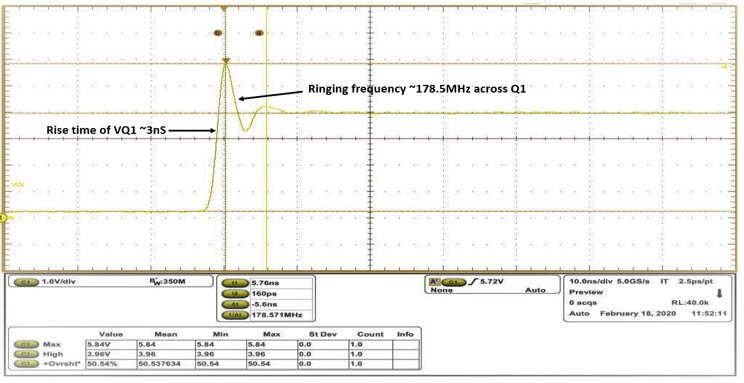Figure 9. PCB layout of the MAX38643 board-2 EV kit layout.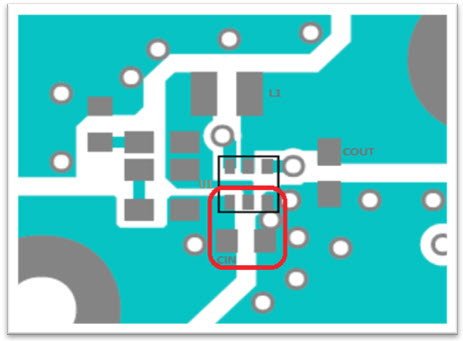Figure 10. Radiated emission peak plot of the MAX38643 board-2 EV kit layout.

The radiated EMI emission is reduced by placing CIN near the IC MAX38643 (Figure 10). The EMI emission is reduced by about more than 4dBµV/m to 5dBµV/m from frequency 300MHz to 350MHz. The board-2 layout has reduced EMI emissions. Further improvements can be made by adding a RC snubber across Q1 or Q2.

#### Benefits of the RC Snubber Circuit

The MAX3864x nano-power buck converter family has MOSFETs Q1 and Q2 integrated within the chip. The EMI noise is generated by the high dv/dt, di/dt in the circuit, which in turn causes the ringing in the voltage across Q1 and Q2 during high frequency switching. The analysis of Figure 5 shows the parasitic inductance is more from the CIN to Q1 drain terminal due to the trace on the PCB, and less from the Q1 source terminal to Q2 drain terminal as both Q1 and Q2 are inside the chip. Hence, the ringing voltage across Q1 is more than Q2. Figures 11a and 11b are the voltage waveforms across Q1 and Q2 in the board-2 PCB layout.Figure 11a. Voltage across Q1.Figure 11b: Voltage across Q2.

A RC snubber is applied across Q1 to reduce the radiated EMI noise as the ringing is high across Q1 (Figure 12).Figure 12. Buck converter with RC snubber across switch Q1.

#### Calculation of the RC Snubber

Let us calculate the parasitic inductance and capacitance in the circuit which leads to the radiated EMI emission. The waveform in Figure 13 shows the voltage across Q1 during the OFF condition in the board-2 PCB layout.Figure 13. Voltage across switch Q1 of the MAX38643 board-2 EV kit layout.

The ringing voltage across Q1 has a frequency of 185.1MHz, which is the result of parasitic inductance and capacitance (Figure 13). The parasitic capacitance is calculated by increasing the capacitance across Q1 until the ringing frequency reduces to half the value. The resonance frequency is inversely proportionate to the square root of the capacitance for the same inductance value.

The frequency of the ringing decreases from 185.1MHz to 94.3MHz by adding the capacitance of 820pF across Q1. Figure 14 shows the voltage waveform across Q1.Figure 14. Voltage across the switch Q1 with 820pF.

The parasitic capacitance can now be calculated by below equation 1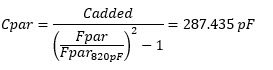(Equation 1)

Where,
Fpar = 185.1 MHz,
Fpar_820pF = 94.3 MHz

The parasitic inductance is calculated as(Equation 2)

The snubber resistor is now calculated as: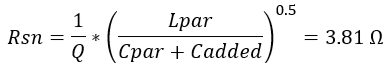(Equation 3)

Where, Q=0.4.
The selected Rsn value is, Rsn=4.7?

Figure 15 shows the waveform when the 4.7?, 820pF snubber values are connected across Q1. The ringing voltage reduces to 4.68V peak. The rise time of VQ1 increases from 3nS to 3.2nS.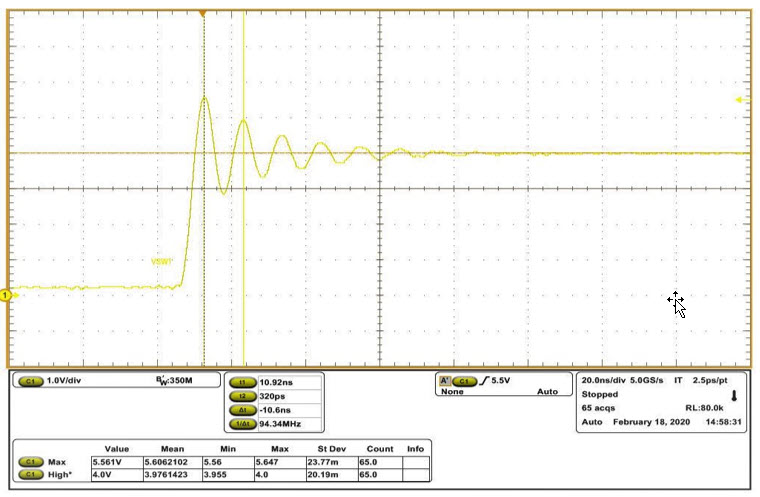Figure 15. Voltage across the switch Q1 with 4.7?, 820pF.

#### Optimizing the RC Snubber for Efficiency

The calculation of the RC snubber and damping of the ringing voltage across Q1 is already demonstrated. But the RC snubber increases the power loss in the converter, and this increased power loss in the circuit is wasted in the RC snubber itself. It is very important to set the RC snubber by keeping the efficiency in mind. Multiple snubber values have been tried to see the effects on the efficiency and ringing voltage of the switching node across Q1. Tables 2, 3, and 4 shows the efficiency results for the board-2 layout without the snubber and with different RC snubbers across Q1. Figure 16 shows the schematic of the buck converter with a snubber added across Q1.

#### Efficiency results

Table 2: Efficiency Data of MAX38643 on the Board-2 EV Kit.

MAX38643 - BOARD-2 EV KIT
Vin (V) Iin (mA) Vo (V) Io (mA) Eff (%)Vreg (%)
4.0 5.29 1.82 10.19 87.6 1.11
4.0 51.44 1.80 100.83 88.2 -0.04
4.0 251.22 1.79 504.23 89.6 -0.72

Table 3: Efficiency Data of MAX38643 with a (4.7? + 820pF) RC Snubber across Q1.

MAX38643 - BOARD-2 EV KIT WITH RC SNUBBER (4.7? + 820pF) ACROSS Q1
Vin (V) Iin (mA) Vo (V) Io (mA) Eff (%)Vreg (%) Diff Eff (%)
4.0 5.33 1.82 10.02 85.5 1.12 2.1
4.0 52.67 1.80 100.72 86.1 -0.03 2.1
4.0 258.99 1.79 508.21 87.5 -0.77 2.1
4.0 365.00 1.78 707.45 86.3 -1.13 1.7

Table 4: Efficiency Data of MAX38643 with a (4.7? + 47pF) RC Snubber across Q1.

MAX38643 - Board-2 EV Kit with RC snubber (4.7?+47pF) across Q1
Vin (V) Iin (mA) Vo (V) Io (mA) Eff (%)Vreg (%) Diff Eff (%)
4.0 5.27 1.82 10.14 87.5 1.11 0.1
4.0 51.27 1.80 100.34 88.0 -0.04 0.1
4.0 252.21 1.79 505.34 89.5 -0.74 0.1
4.0 355.67 1.78 701.87 87.8 -1.09 0.2Figure 16. MAX38643 buck converter with a RC snubber (R2, C4) across the switch Q1.

#### Effect of the RC Snubber on EMI Emission

The effects of different RC snubbers on the efficiency and ringing voltage across Q1 is already demonstrated. The snubber values of 4.7? and 47pF were selected to create less loss and achieve high efficiency in the converter, and to keep the noise level much below the Class B limit. Figures 17 and 18 show the Q1 voltage waveform, and EMI emission with a 4.7? and 47pF snubber across Q1.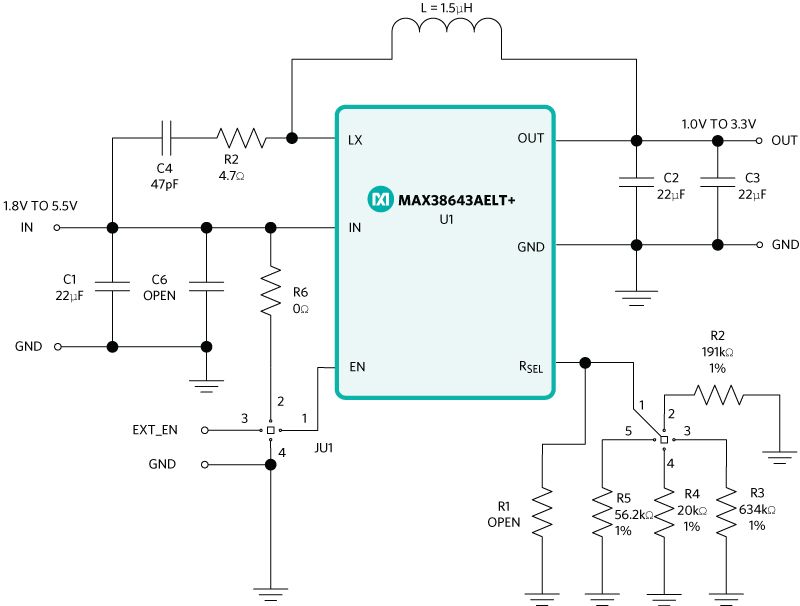Figure 17. Voltage across the switch Q1 with 4.7?/47pF.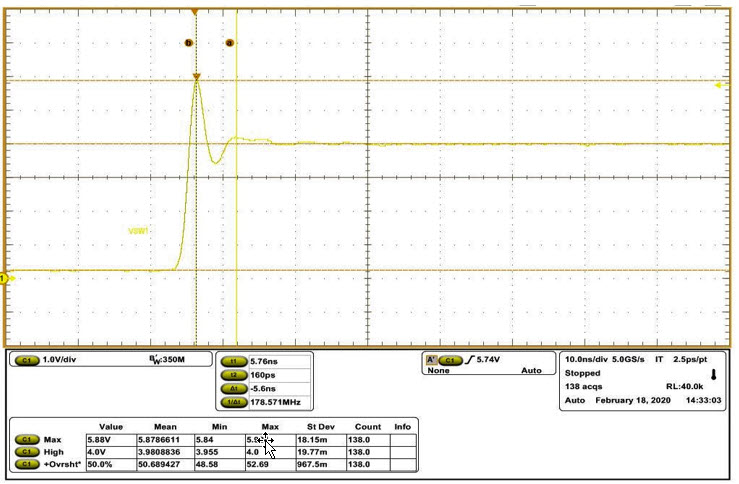Figure 18. Radiated EMI peak plot of the MAX38643 board-2 EV kit layout with a 4.7?/47pF snubber across Q1.

The selected snubber values achieved more than a 6dBµV/m margin from the CISPR32 standard (Figure 18).

#### Radiated EMI Results of the MAX38643AELT+ Buck Converter

Figures 19a and 19b show the MAX38643 buck converter-radiated EMI results on board-1 and board-2 with a RC snubber across Q1. The tests were performed in a third-party certified 10-meter EMI chamber. The test results show that board-1 passed the CISPR32 Class B limit with a 2.2dB margin. The board-2 with a RC snubber passed the CISPR32 Class B limit with more than a 6dB margin without compromising the efficiency.Figure 19a. Radiated EMI result of the MAX38643 board-1 EV kit layout.Figure 19b. Radiated EMI result of the MAX38643 board-2 EV kit with a 4.7?/47pF snubber across Q1.

#### Conclusion

This application note first measures the radiated EMI emission of the MAX38643 EV kit in a 10-meter chamber. The test result passes the CISPR32 Class B limit with very less margin. The EMI emission improves with the improvements in the PCB layout. A proper RC snubber to reduce the noise helps pass the CISPR32 Class B limit with more than a 6dB margin without compromising the efficiency in the MAX38643 buck converter.